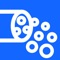## Darcy-Weisbach Equations Calculator are physic/math calculator to find fluid head loss, friction factor, pipe length, pipe diameter or fluid flow velocity fast and easy# Darcy Weisbach Equations Calc

by NitrioDarcy-Weisbach Equations Calculator are physic/math calculator to find fluid head loss, friction factor, pipe length, pipe diameter or fluid flow velocity fast and easy.### App Details

Version
1.1
Rating
NA
Size
8Mb
Genre
Utilities Productivity
Last updated
November 9, 2020
Release date
January 12, 2019

### App Screenshots### App Store Description

Darcy-Weisbach Equations Calculator are physic/math calculator to find fluid head loss, friction factor, pipe length, pipe diameter or fluid flow velocity fast and easy.

Features:
- Instant calculation
- Result are copy able to other app
- Formula are include as reference
- Support up to 16 decimal place
- Support various unit for each input

Darcy-Weisbach Equations for Hydraulics Fluid Mechanics
In fluid dynamics, the Darcy–Weisbach equation is an empirical equation, which relates the head loss, or pressure loss, due to friction along a given length of pipe to the average velocity of the fluid flow for an incompressible fluid. The equation is named after Henry Darcy and Julius Weisbach.

Darcy-Weisbach equation are used to calculate the major pressure or head loss due to friction in pipes, ducts or tubes

Formula:

h = fLv²/2Dg

Where: